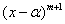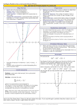How to Learn in 24 Hours?The Rapid Learning Movie

 Need Help? M-F: 9am-5pm(PST): Toll-Free: (877) RAPID-10 US Direct: (714) 692-2900 Int'l: 001-714-692-2900 24/7 Online Technical Support: The Rapid Support Center Secure Online Order:Got Questions? Frequently Asked Questions
 Need Proof? Testimonials by Our Users
 Trustlink is a Better Business Bureau Program. Rapid Learning Center is a fivr-star business. External TrustLink Reviews

 Rapid Learning Courses: MCAT in 24 Hours (2015-16) USMLE in 24 Hours (Boards) Chemistry in 24 Hours Biology in 24 Hours Physics in 24 Hours Mathematics in 24 Hours Psychology in 24 Hours SAT in 24 Hours ACT in 24 Hours AP in 24 Hours CLEP in 24 Hours DAT in 24 Hours (Dental) OAT in 24 Hours (Optometry) PCAT in 24 Hours (Pharmacy) Nursing Entrance Exams Certification in 24 Hours eBook - Survival Kits Audiobooks (MP3)

 Tell-A-Friend: Have friends taking science and math courses too? Tell them about our rapid learning system.Home »  Mathematics »  College Algebra

Zeros of Polynomial Functions

 Topic Review on "Title": Root: A root is any real or complex number a where a polynomial takes the value 0. Multiplicity of a Root: The multiplicity of a rootof a polynomial f(x) has multiplicity m ifdivides f(x) anddoes not divide f(x). Rolle’s Theorem: Let f(x) be a polynomial and a and b are two numbers such that a

Rapid Study Kit for "Title":
 Flash Movie Flash Game Flash Card Core Concept Tutorial Problem Solving Drill Review Cheat Sheet"Title" Tutorial Summary : This tutorial shows the basic definitions of the zeros of polynomial functions. Quadratic and cubic polynomials are presented with the use of graphs and the quadratic equation. The nature of roots is shown through the examples in this tutorial. Polynomials of higher degree are shown with the use of the rational test, and Rolle’s Theorem. The roots and factoring polynomials are mentioned using theorems such as the irreducibility criterion and the fundamental theorem of algebra. Finally, an introduction to mathematical modeling is presented with the least squares principles and the regression model.

 Tutorial Features: Specific Tutorial Features: • Examples showing how the roots of polynomials are found in some cases. • Graphical representation of the regression and least squares principles to emphasize the theory of mathematical modeling. Series Features: • Concept map showing inter-connections of new concepts in this tutorial and those previously introduced. • Definition slides introduce terms as they are needed. • Visual representation of concepts • Animated examples—worked out step by step • A concise summary is given at the conclusion of the tutorial.

 "Title" Topic List: Basic definitions of the zeros of polynomial functionsQuadratic and Cubic PolynomialsPolynomials of higher degree Rolle’s Theorem The Rational Root Test Descartes RuleRoots and Factoring Irreducibility criterion The Fundamental Theorem of AlgebraMathematical Modeling Regression PrincipleLeast Squares Principle

See all 24 lessons in College Algebra, including concept tutorials, problem drills and cheat sheets:
Teach Yourself College Algebra Visually in 24 Hours